## Logistic growth

Difference equation : the following forms are equivalent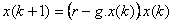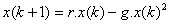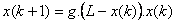The interactive program below produces Logistic Growth. Input the initial value x(0) and constant r and g then click "Find" button to find x(n) and to show the plot.

Initial value x(0) = Constant r = > 1 Constant g = Find the value of x at n =

Characteristics of Logistic Growth Model:

• Multiply by a growth factor that depends linearly on the current terms of the sequence
• The growth factor is linearly depend on the size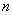•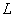= capacity.
• If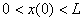:
• If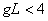, then every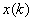is remain in the interval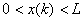• If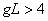, then the model may produce unrealistic results such as negativeor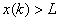• If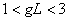, the model will eventually level off at a steady value of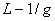.
• If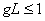, thewill steadily decrease with zero asymptote. If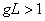, thewill level off to a steady positive value of